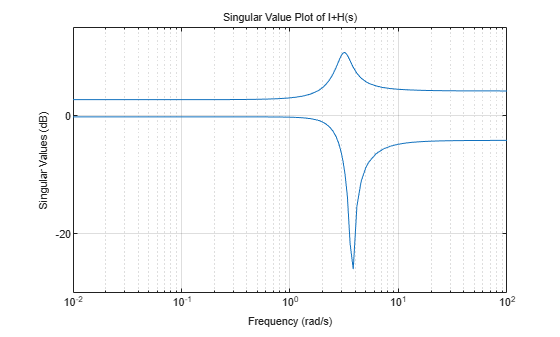# sigmaoptions

Create list of sigma plot options

## Description

Use the `sigmaoptions` command to create a `SigmaPlotOptions` object to customize your sigma plot appearance. You can also use the command to override the plot preference settings in the MATLAB® session in which you create the sigma plots.

## Creation

### Syntax

``plotoptions = sigmaoptions``
``plotoptions = sigmaoptions('cstprefs')``

### Description

example

````plotoptions = sigmaoptions` returns a default set of plot options for use with the `sigmaplot` command. You can use these options to customize the sigma plot appearance using the command line. This syntax is useful when you want to write a script to generate plots that look the same regardless of the preference settings of the MATLAB session in which you run the script.```

example

````plotoptions = sigmaoptions('cstprefs')` initializes the plot options with the options you selected in the Control System Toolbox™ Preferences Editor. For more information about the editor, see Toolbox Preferences Editor. This syntax is useful when you want to change a few plot options but otherwise use your default preferences. A script that uses this syntax may generate results that look different when run in a session with different preferences.```

## Properties

expand all

Frequency units, specified as one of the following values:

• `'Hz'`

• `'rad/s'`

• `'rpm'`

• `'kHz'`

• `'MHz'`

• `'GHz'`

• `'rad/nanosecond'`

• `'rad/microsecond'`

• `'rad/millisecond'`

• `'rad/minute'`

• `'rad/hour'`

• `'rad/day'`

• `'rad/week'`

• `'rad/month'`

• `'rad/year'`

• `'cycles/nanosecond'`

• `'cycles/microsecond'`

• `'cycles/millisecond'`

• `'cycles/hour'`

• `'cycles/day'`

• `'cycles/week'`

• `'cycles/month'`

• `'cycles/year'`

Frequency scale, specified as either '`log`' or '`linear`' .

Magnitude units, specified as either '`dB`' or absolute value '`abs`'.

Magnitude scale, specified as either '`log`' or '`linear`'.

Grouping of input-output (I/O) pairs, specified as one of the following:

• '`none`' — No input-output grouping.

• '`inputs`' — Group only the inputs.

• '`outputs`' — Group only the outputs.

• '`all`' — Group all the I/O pairs.

Input label style, specified as a structure with the following fields:

• `FontSize` — Font size, specified as a scalar value greater than zero in point units. The default font size depends on the specific operating system and locale. One point equals `1/72` inch.

• `FontWeight` — Character thickness, specified as '`Normal`' or '`bold`'. MATLAB uses the `FontWeight` property to select a font from those available on your system. Not all fonts have a bold weight. Therefore, specifying a bold font weight can still result in the normal font weight.

• `FontAngle` — Character slant, specified as '`Normal`' or '`italic`'. Not all fonts have both font styles. Therefore, the italic font might look the same as the normal font.

• `Color` — Text color, specified as an RGB triplet. The default color is dark grey with the RGB triplet `[0.4,0.4,0.4]`.

• `Interpreter` — Text interpreter, specified as one of these values:

• '`tex`' — Interpret characters using a subset of TeX markup. This is the default value of `Interpreter`.

• '`latex`' — Interpret characters using LaTeX markup.

• '`none`' — Display literal characters.

Output label style, specified as a structure with the following fields:

• `FontSize` — Font size, specified as a scalar value greater than zero in point units. The default font size depends on the specific operating system and locale. One point equals `1/72` inch.

• `FontWeight` — Character thickness, specified as '`Normal`' or '`bold`'. MATLAB uses the `FontWeight` property to select a font from those available on your system. Not all fonts have a bold weight. Therefore, specifying a bold font weight can still result in the normal font weight.

• `FontAngle` — Character slant, specified as '`Normal`' or '`italic`'. Not all fonts have both font styles. Therefore, the italic font might look the same as the normal font.

• `Color` — Text color, specified as an RGB triplet. The default color is dark grey with the RGB triplet `[0.4,0.4,0.4]`.

• `Interpreter` — Text interpreter, specified as one of these values:

• '`tex`' — Interpret characters using a subset of TeX markup. This is the default value of `Interpreter`.

• '`latex`' — Interpret characters using LaTeX markup.

• '`none`' — Display literal characters.

Toggle display of inputs, specified as either `{'on'}`, `{'off'}` or a cell array with multiple elements.

Toggle display of outputs, specified as either `{'on'}`, `{'off'}` or a cell array with multiple elements.

Title text and style, specified as a structure with the following fields:

• `String` — Label text, specified as a character vector. By default, the plot is titled `'Singular Values'`.

• `FontSize` — Font size, specified as a scalar value greater than zero in point units. The default font size depends on the specific operating system and locale. One point equals `1/72` inch.

• `FontWeight` — Character thickness, specified as '`Normal`' or '`bold`'. MATLAB uses the `FontWeight` property to select a font from those available on your system. Not all fonts have a bold weight. Therefore, specifying a bold font weight can still result in the normal font weight.

• `FontAngle` — Character slant, specified as '`Normal`' or '`italic`'. Not all fonts have both font styles. Therefore, the italic font might look the same as the normal font.

• `Color` — Text color, specified as an RGB triplet. The default color is black specified by the RGB triplet `[0,0,0]`.

• `Interpreter` — Text interpreter, specified as one of these values:

• '`tex`' — Interpret characters using a subset of TeX markup. This is the default value of `Interpreter`.

• '`latex`' — Interpret characters using LaTeX markup.

• '`none`' — Display literal characters.

X-axis label text and style, specified as a structure with the following fields:

• `String` — Label text, specified as a character vector. By default, the axis is titled based on the frequency units `FreqUnits`.

• `FontSize` — Font size, specified as a scalar value greater than zero in point units. The default font size depends on the specific operating system and locale. One point equals `1/72` inch.

• `FontWeight` — Character thickness, specified as '`Normal`' or '`bold`'. MATLAB uses the `FontWeight` property to select a font from those available on your system. Not all fonts have a bold weight. Therefore, specifying a bold font weight can still result in the normal font weight.

• `FontAngle` — Character slant, specified as '`Normal`' or '`italic`'. Not all fonts have both font styles. Therefore, the italic font might look the same as the normal font.

• `Color` — Text color, specified as an RGB triplet. The default color is black specified by the RGB triplet `[0,0,0]`.

• `Interpreter` — Text interpreter, specified as one of these values:

• '`tex`' — Interpret characters using a subset of TeX markup. This is the default value of `Interpreter`.

• '`latex`' — Interpret characters using LaTeX markup.

• '`none`' — Display literal characters.

Y-axis label text and style, specified as a structure with the following fields:

• `String` — Label text, specified as a cell array of character vectors. By default, the axis is titled based on the magnitude units `MagUnits`.

• `FontSize` — Font size, specified as a scalar value greater than zero in point units. The default font size depends on the specific operating system and locale. One point equals `1/72` inch.

• `FontWeight` — Character thickness, specified as '`Normal`' or '`bold`'. MATLAB uses the `FontWeight` property to select a font from those available on your system. Not all fonts have a bold weight. Therefore, specifying a bold font weight can still result in the normal font weight.

• `FontAngle` — Character slant, specified as '`Normal`' or '`italic`'. Not all fonts have both font styles. Therefore, the italic font might look the same as the normal font.

• `Color` — Text color, specified as an RGB triplet. The default color is black specified by the RGB triplet `[0,0,0]`.

• `Interpreter` — Text interpreter, specified as one of these values:

• '`tex`' — Interpret characters using a subset of TeX markup. This is the default value of `Interpreter`.

• '`latex`' — Interpret characters using LaTeX markup.

• '`none`' — Display literal characters.

Tick label style, specified as a structure with the following fields:

• `FontSize` — Font size, specified as a scalar value greater than zero in point units. The default font size depends on the specific operating system and locale. One point equals `1/72` inch.

• `FontWeight` — Character thickness, specified as '`Normal`' or '`bold`'. MATLAB uses the `FontWeight` property to select a font from those available on your system. Not all fonts have a bold weight. Therefore, specifying a bold font weight can still result in the normal font weight.

• `FontAngle` — Character slant, specified as '`Normal`' or '`italic`'. Not all fonts have both font styles. Therefore, the italic font might look the same as the normal font.

• `Color` — Text color, specified as an RGB triplet. The default color is black specified by the RGB triplet `[0,0,0]`.

Toggle grid display on the plot, specified as either '`off`' or '`on`'.

Color of the grid lines, specified as an RGB triplet. The default color is light grey specified by the RGB triplet `[0.15,0.15,0.15]`.

Selection mode for the x-axis limits, specified as one of these values:

• '`auto`' — Enable automatic limit selection, which is based on the total span of the plotted data.

• '`manual`' — Manually specify the axis limits. To specify the axis limits, set the `XLim` property.

Selection mode for the y-axis limits, specified as one of these values:

• '`auto`' — Enable automatic limit selection, which is based on the total span of the plotted data.

• '`manual`' — Manually specify the axis limits. To specify the axis limits, set the `YLim` property.

X-axis limits, specified as a cell array of two-element vector of the form `[min,max]`.

Y-axis limits, specified as a cell array of two-element vector of the form `[min,max]`.

## Object Functions

 `sigmaplot` Plot singular values of frequency response with additional plot customization options

## Examples

collapse all

For this example, use the plot handle to change the frequency units to Hz and turn on the grid.

Generate a random state-space model with 5 states and create the sigma plot with plot handle `h`.

```rng("default") sys = rss(5); h = sigmaplot(sys);```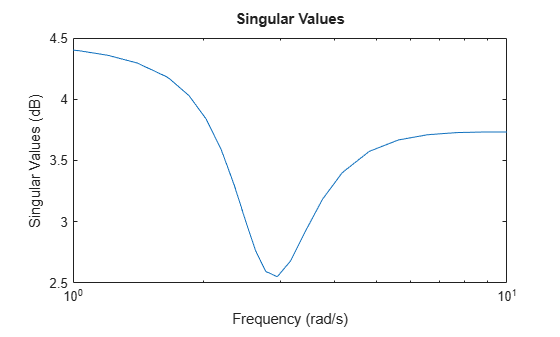Change the units to Hz and turn on the grid. To do so, edit properties of the plot handle, `h` using `setoptions`.

`setoptions(h,'FreqUnits','Hz','Grid','on');`The sigma plot automatically updates when you call `setoptions`.

Alternatively, you can also use the `sigmaoptions` command to specify the required plot options. First, create an options set based on the toolbox preferences.

`p = sigmaoptions('cstprefs');`

Change properties of the options set by setting the frequency units to Hz and enable the grid.

```p.FreqUnits = 'Hz'; p.Grid = 'on'; sigmaplot(sys,p);```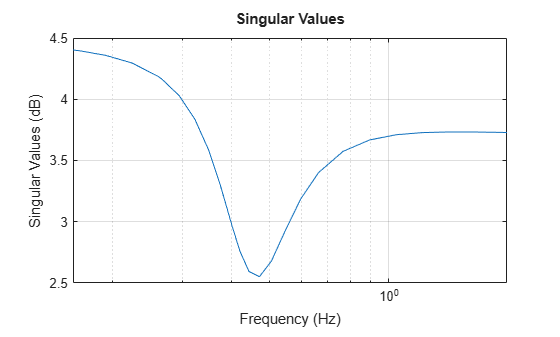You can use the same option set to create multiple sigma plots with the same customization. Depending on your own toolbox preferences, the plot you obtain might look different from this plot. Only the properties that you set explicitly, in this example `Grid` and `FreqUnits`, override the toolbox preferences.

For this example, create a sigma plot that uses 15-point red text for the title. This plot should look the same, regardless of the preferences of the MATLAB session in which it is generated.

First, create a default options set using `sigmaoptions`.

`plotoptions = sigmaoptions;`

Next, change the required properties of the options set `plotoptions`.

```plotoptions.Title.FontSize = 15; plotoptions.Title.Color = [1 0 0]; plotoptions.FreqUnits = 'Hz'; plotoptions.Grid = 'on';```

Now, create a sigma plot using the options set `plotoptions`.

`h = sigmaplot(tf(1,[1,1]),plotoptions);`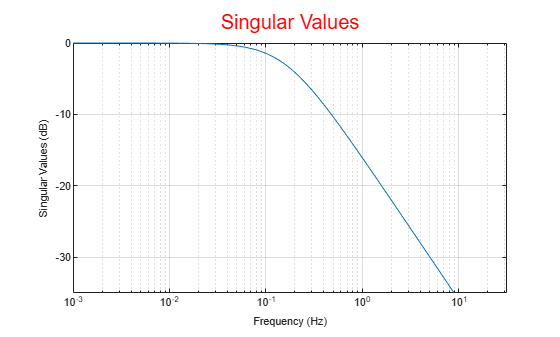Because `plotoptions` begins with a fixed set of options, the plot result is independent of the toolbox preferences of the MATLAB session.

For this example, create a sigma plot of the following continuous-time SISO dynamic system. Then, turn the grid on, rename the plot and change the frequency scale.

`$sys\left(s\right)=\frac{{s}^{2}+0.1s+7.5}{{s}^{4}+0.12{s}^{3}+9{s}^{2}}.$`

Create the transfer function `sys`.

`sys = tf([1 0.1 7.5],[1 0.12 9 0 0]);`

Next, create the options set using `sigmaoptions` and change the required plot properties.

```plotoptions = sigmaoptions; plotoptions.Grid = 'on'; plotoptions.FreqScale = 'linear'; plotoptions.Title.String = 'Singular Value Plot of Transfer Function';```

Now, create the sigma plot with the custom option set `plotoptions`.

`h = sigmaplot(sys,plotoptions);`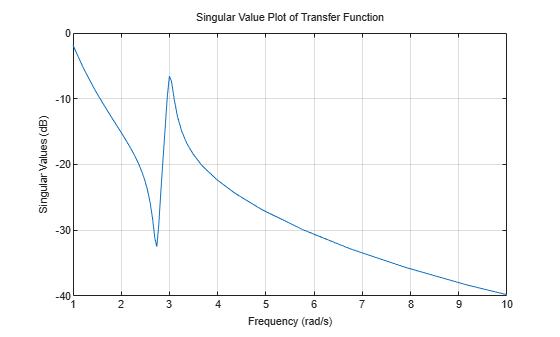`sigmaplot` automatically selects the plot range based on the system dynamics.

For this example, compare the SV for the frequencies of a parametric model, identified from input/output data, to a non-parametric model identified using the same data. Identify parametric and non-parametric models based on the data.

Load the data and create the parametric and non-parametric models using `tfest` and `spa`, respectively.

```load iddata2 z2; w = linspace(0,10*pi,128); sys_np = spa(z2,[],w); sys_p = tfest(z2,2);```

`spa` and `tfest` require System Identification Toolbox™ software. The model `sys_np` is a non-parametric identified model while, `sys_p` is a parametric identified model.

Create an options set to turn the grid on. Then, create a sigma plot that includes both systems using this options set.

```plotoptions = sigmaoptions; plotoptions.Grid = 'on'; h = sigmaplot(sys_p,'b--',sys_np,'r--',w,plotoptions); legend('Parametric Model','Non-Parametric model');```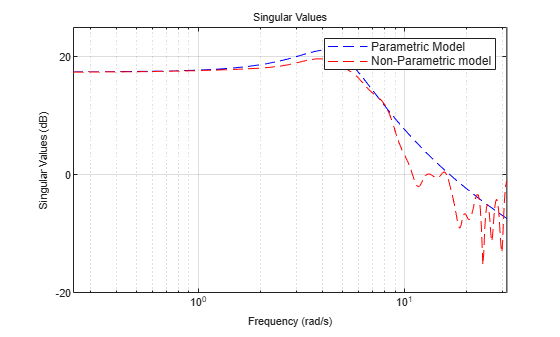Consider the following two-input, two-output dynamic system.

`$H\left(s\right)=\left[\begin{array}{cc}0& \frac{3s}{{s}^{2}+s+10}\\ \frac{s+1}{s+5}& \frac{2}{s+6}\end{array}\right].$`

Plot the singular value responses of H(s) and I + H(s). Set appropriate titles using the plot option set.

```H = [0, tf([3 0],[1 1 10]) ; tf([1 1],[1 5]), tf(2,[1 6])]; opts1 = sigmaoptions; opts1.Grid = 'on'; opts1.Title.String = 'Singular Value Plot of H(s)'; h1 = sigmaplot(H,opts1);```Use input 2 to plot the modified SV of type, I + H(s).

```opts2 = sigmaoptions; opts2.Grid = 'on'; opts2.Title.String = 'Singular Value Plot of I+H(s)'; h2 = sigmaplot(H,[],2,opts2);```